Next: Controlling the angle Up: Theory review Previous: Theory review

## Redefining R

The principle of causality: no response before a stimulus, is not considered in the R definition [equation (2)]. The omission of causality translates into improper behavior of the high frequencies. In order to include causality into the definition of R, let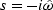be the causal, positive, discrete representation of the differentiation operator,(3)

which is simplified by writting.

Claerbout (1985) proposes the use of the following Muir recursion starting from R0=s:(4)

This recursion produces a continuous fraction. Studying the limit for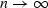we obtain: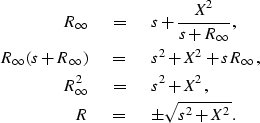(5)

If we let X2 = v2 kx2 in equation (5) then R is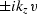. The retarded time expression of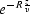[equation (6)] will downward continue, in time, the data for phase-shift migration.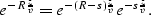(6)

The following change of variables: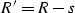(7)

transforms the Muir recursion (4) into:(8)

Again, taking the limit forin this recursion we will obtain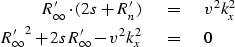(9)

This quadratic expression yields to two square roots for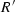,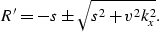(10)

We need to select the one that is able to handle the evanescent region, i.e, the square root that goes to zero at kx = 0, which corresponds to the positive square root.(11)
multiplying numerator and denominator by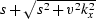, equation (11) transforms into: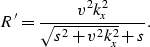(12)

I already showed that the real part ofis positive (Figure 1); therefore,, as defined in equation (12), also has a positive real part.

Finally, we downward continue the data in the Fourier domain by multiplying by: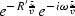(13)

Expression (13) incorporates the causality and viscosity concepts in phase shift migration, controls the evanescent energy and will not allow discontinuity between evanescent and non-evanescent regions.Next: Controlling the angle Up: Theory review Previous: Theory review
Stanford Exploration Project
4/29/2001